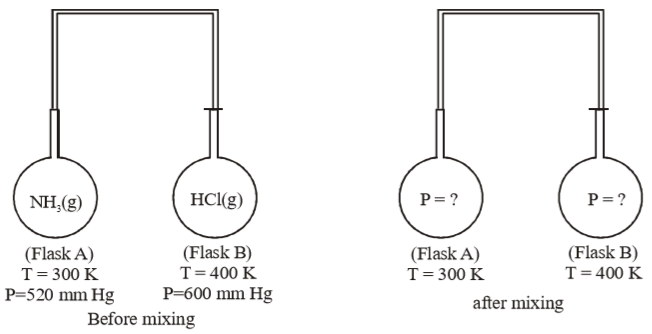Two vessels of capacities 3 litres and 4 litres are separately filled with a gas. The pressures are respectively 202 kPa and 101 kPa. The two vessels are connected. The gas pressure will be now, at constant temperature.

(A) 151.5 kPa

(B) 144 kPa

(C) 303 kPa

(D) 175 kPa

High Yielding Test Series + Question Bank - NEET 2020

Difficulty Level:

2 gms of hydrogen diffuses from a container in 10 minutes. How many gms of oxygen would diffuse through the same time under similar conditions ?

(A) 0.5 gm

(B) 4 gm

(C) 6 gm

(D) 8 gm

Concept Questions :-

Graham's Law
High Yielding Test Series + Question Bank - NEET 2020

Difficulty Level:

Which of the following contains the greatest number of nitrogen atoms ?

(A) 500 ml of 2.0

(B) One mole of ${\mathrm{NH}}_{4}\mathrm{Cl}$

(C)  molecules of ${\mathrm{NO}}_{2}$ gas

(D) 22.4 litres of ${\mathrm{N}}_{2}$ gas at 0ºC and 1 atm.

Concept Questions :-

Introduction to States of Matter
High Yielding Test Series + Question Bank - NEET 2020

Difficulty Level:

The temperature of a sample of gas is raised from 127ºC to 527ºC. The average kinetic energy of the gas$-$

(A) Does not changes

(B) Is doubled

(C) Is halved

(D) Cannot be calculated

Concept Questions :-

Kinetic Theory of Gas
High Yielding Test Series + Question Bank - NEET 2020

Difficulty Level:

A helium atom is two times heavier than a hydrogen molecule at 298 K, the average kinetic energy of helium is

(A) Two times that of hydrogen molecules

(B) Same as that of hydrogen molecules

(C) Four times that of hydrogen molecules

(D) Half that of hydrogen molecules

Concept Questions :-

Kinetic Theory of Gas
High Yielding Test Series + Question Bank - NEET 2020

Difficulty Level:

Two flasks A and B of equal volume containing ${\mathrm{NH}}_{3}$ and HCl gases, are connected by a narrow tube of negligible volume. The two gases were prevented from mixing by stopper fitted in connecting tube. For further detail of experiment refer to the given figure. What will be final pressure in each flask when passage connecting two tubes are opened. Assume ideal gas behaviour of ${\mathrm{NH}}_{3}$ and $\mathrm{HCl}$ gas and the reaction.(A) 40 mm Hg

(B) 60 mm Hg

(C) 20 mm Hg

(D) 10 mm Hg

Concept Questions :-

Dalton's Law
High Yielding Test Series + Question Bank - NEET 2020

Difficulty Level:

The ratio of average molecular kinetic energy of ${\mathrm{UF}}_{6}$ to that of ${\mathrm{H}}_{2}$, both at 300 K is$-$

(A) 1 : 1

(B) 7 : 2

(C) 176 : 1

(D) 2 : 7

Concept Questions :-

Molecular Velocity
High Yielding Test Series + Question Bank - NEET 2020

Difficulty Level:

A mono atomic gas diatomic gas and triatomic gas are mixed, taking one mole of each ${\mathrm{C}}_{\mathrm{p}}/{\mathrm{C}}_{\mathrm{v}}$ for the mixture is$-$

(A) 1.40

(B) 1.428

(C) 1.67

(D) 1.33

Concept Questions :-

Kinetic Theory of Gas
High Yielding Test Series + Question Bank - NEET 2020

Difficulty Level:

According to kinetic theory of gases, for a diatomic molecule

(A) The pressure exerted by the gas is proportional to the mean velocity of the molecule.

(B) The pressure exerted by the gas is proportional to the root mean square velocity of the molecule.

(C) The root mean square velocity of the molecule is inversely proportional to the temperature.

(D) The mean translational kinetic energy of the molecule is proportional to the absolute temperature.

Concept Questions :-

Kinetic Theory of Gas
High Yielding Test Series + Question Bank - NEET 2020

Difficulty Level:

The values of vander waals constant ‘a’ for the gases ${\mathrm{O}}_{2}$, ${\mathrm{N}}_{2}$${\mathrm{NH}}_{3}$ and ${\mathrm{CH}}_{4}$ are 1.36, 1.39, 4.17 and 2.253 ${\mathrm{lit}}^{2}$ atom mol-2 respectively. The gas which can most easily be liquefied is$-$

(A) ${\mathrm{O}}_{2}$

(B) ${\mathrm{N}}_{2}$

(C) ${\mathrm{NH}}_{3}$

(D) ${\mathrm{CH}}_{4}$

Concept Questions :-

Vanderwaal Correction